Courses

# Geology - 2016 Past Year Paper

## 60 Questions MCQ Test IIT JAM Past Year Papers and Model Test Paper (All Branches) | Geology - 2016 Past Year Paper

Description
This mock test of Geology - 2016 Past Year Paper for IIT JAM helps you for every IIT JAM entrance exam. This contains 60 Multiple Choice Questions for IIT JAM Geology - 2016 Past Year Paper (mcq) to study with solutions a complete question bank. The solved questions answers in this Geology - 2016 Past Year Paper quiz give you a good mix of easy questions and tough questions. IIT JAM students definitely take this Geology - 2016 Past Year Paper exercise for a better result in the exam. You can find other Geology - 2016 Past Year Paper extra questions, long questions & short questions for IIT JAM on EduRev as well by searching above.
QUESTION: 1

Solution:
QUESTION: 2

Solution:
QUESTION: 3

### In the topographic map the steepest slope is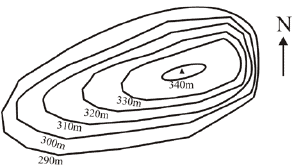Solution:
QUESTION: 4

Gabbro contains much more alumina than peridotite because it is richer in

Solution:
QUESTION: 5

Coking coal in India is found in

Solution:
QUESTION: 6

The amplitude of ground motion during an earthquake of magnitude 7 in Richter scale is how many times more than that of a magnitude 5?

Solution:
QUESTION: 7

The failed arm of a continental rift is called

Solution:
QUESTION: 8

The hardest mineral (with the exception of diamond) in the Moh’s scale of hardness is

Solution:
QUESTION: 9

Which one of the following is capable of transporting sediments against the slope?

Solution:
QUESTION: 10

Which is the most abundant ion in the normal seawater?

Solution:
QUESTION: 11

Match minerals in Group I with the corresponding silicate structure in Group II.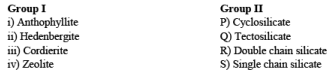Solution:
QUESTION: 12

The correct name for the well sorted sandstone, whose framework composition plots at Y in the following QFR diagram, is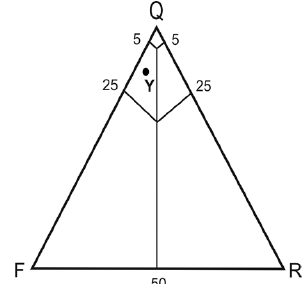Solution:
QUESTION: 13

Which one of the following optical properties of minerals is NOT observed under crossed nicols?

Solution:
QUESTION: 14

Choose the correct stereographic projection among the following that represents 222 crystal symmetry.

Solution:
QUESTION: 15

Match the folds listed in Group I with corresponding geometric characteristics in Group II.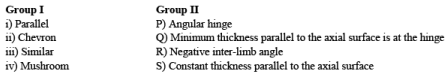Solution:
QUESTION: 16

The number of 4th order stream(s) present in the drainage network shown below, as per hierarchical classification, is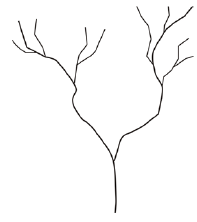Solution:
QUESTION: 17

During metamorphism, temperature can increase at constant pressure in the case of

Solution:
QUESTION: 18

Choose the correct chronological order from oldest to youngest for the following stratigraphic units P - Chitradurga Group; Q - Sargur Group; R - Ajabgarh Group;  S - Udaipur Group

Solution:
QUESTION: 19

Identify the rock types at X and Y in the following QAP diagram of IUGS.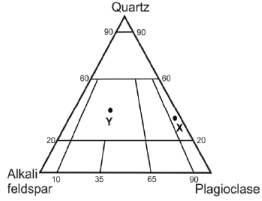Solution:
QUESTION: 20

A schematic diagram of a divergent plate boundary, with arrows indicating directions of plate movement, is given below. Which one of the following statements is NOT true for points P, Q, R and S, if the spreading rate for both the plates is uniform and same through time and space?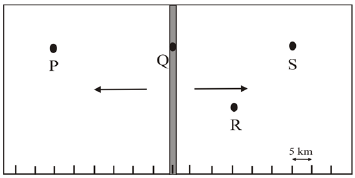Solution:
QUESTION: 21

Mississippian and Pennsylvanian belong to which period?

Solution:
QUESTION: 22

A gravity dam with E–W axis is to be constructed in a narrow river valley between two N–S trending parallel ridges. The river is flowing from south to north. The lithology of the area is represented by 2 to 5 m thick metasedimentary rocks – quartzite, phyllite and schist. Which of the following geological conditions will be most suitable?

Solution:
QUESTION: 23

Match the ore deposits in Group I with the localities in Group II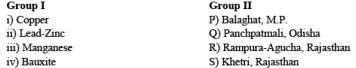Solution:
QUESTION: 24

Match the following stratigraphic units (Group-I) with their ages (Group-II)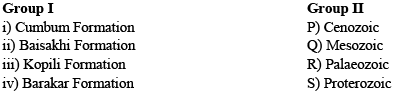Solution:
QUESTION: 25

Which one of the following sedimentary structures is related to gravitational instability?

Solution:
QUESTION: 26

Match features mentioned in Group-I with the fossil types in Group-II.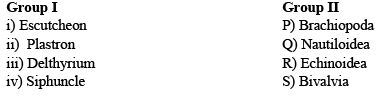Solution:
QUESTION: 27

Which one of the following is an upper Gondwana flora?

Solution:
QUESTION: 28

Choose the correct match of items in Group I with the items in Group II.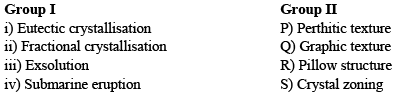Solution:
QUESTION: 29

The fault for which the orientation and displacement vector are given in the following lower hemisphere stereographic projection is a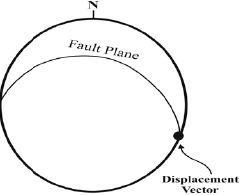Solution:
QUESTION: 30

The change in coordination number of silicon (Si) when α-quartz transforms to stishovite, which has octahedral coordination, is

Solution:
*Multiple options can be correct
QUESTION: 31

Which of the following is/are found only in the brittle shear zones?

Solution:
*Multiple options can be correct
QUESTION: 32

Which among the following statements related to headward erosion by river is/are correct?

Solution:
*Multiple options can be correct
QUESTION: 33

In a thin section of 30 µm thickness, the R.I. of a mineral are: ɛ = 1.565 and ω = 1.468. Choose the correct statement(s) about its optical properties.

Solution:
*Multiple options can be correct
QUESTION: 34

Which of the following statements is/are NOT true for equilibrium crystallization of plagioclase from a melt in the binary system NaAlSi3O8–CaAlSi2O8 at constant pressure?

Solution:
*Multiple options can be correct
QUESTION: 35

The value of gravity at the Earth’s surface is dependent on

Solution:
*Multiple options can be correct
QUESTION: 36

Choose the burrowing form(s) of bivalve.

Solution:
*Multiple options can be correct
QUESTION: 37

Which of the following environments is/are characterized by predominant deposit of mud?

Solution:
*Multiple options can be correct
QUESTION: 38

Which of the following stratigraphic units belong(s) to Cretaceous?

Solution:
*Multiple options can be correct
QUESTION: 39

Which of the following ore deposit(s) is/are formed only by hydrothermal process?

Solution:
*Multiple options can be correct
QUESTION: 40

Migmatite is a rock

Solution:
*Answer can only contain numeric values
QUESTION: 41

Based on the ideal end member formula of diopside, the mole proportion (%) of CaO for plotting the mineral on a CaO-MgO-SiO2 triangular diagram is________.

Solution:
*Answer can only contain numeric values
QUESTION: 42

The phi (ϕ) value of a sediment particle having 4 mm diameter is ________.

Solution:
*Answer can only contain numeric values
QUESTION: 43

Calcite, quartz, wollastonite and CO2 fluid were present in equilibrium during the formation of a calc-silicate rock. In the chemical system CaO–SiO2–CO2, the degree of freedom of this assemblage is _______.

Solution:
*Answer can only contain numeric values
QUESTION: 44

Weight of a10 cm3 medium grained sandstone block with 20% (v/v) porosity, in dry state is 26g. The density of the block when fully saturated with water is _________g/cm3.

Solution:
*Answer can only contain numeric values
QUESTION: 45

In the following figure, the exterior angle measured between (001)∧(021) with a goniometer in a crystal is 40o. The interior angle between (010)∧(021) in degrees is ______.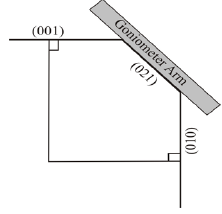Solution:
*Answer can only contain numeric values
QUESTION: 46

If the elevation of a wave cut platform is 55 m above the sea level and the age of the erosional surface is 120 kilo years, the rate of rock uplift at this coastal location is ________m/kilo years (give answer in two decimal places).

Solution:
*Answer can only contain numeric values
QUESTION: 47

A foliation plane has strike 025o and 60o easterly dip. A mineral lineation on this foliation plane has a rake/pitch of 90o. The plunge direction of the mineral lineation in whole circle bearing is _______degrees.

Solution:
*Answer can only contain numeric values
QUESTION: 48

Two outcrops on a 1 : 25000 map are 12 cm apart. The ground distance between the two outcrops is ________ km.

Solution:
*Answer can only contain numeric values
QUESTION: 49

Fine muds are deposited at a rate of 1 cm per 1000 y. Assuming constant sedimentation rate and absence of compaction, a 1 km thick sequence would be deposited in _______million years.

Solution:
*Answer can only contain numeric values
QUESTION: 50

B and B′are two points on the topographic map shown below. The distance between B and B′ along the linear traverse BB′ is 220m. The angle of the slope along this traverse is ________ degree (give answer in two decimal places).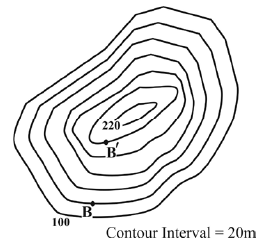Solution:
*Answer can only contain numeric values
QUESTION: 51

The half life of a radionuclide A is double that of a radionuclide B. The fraction of A remaining when B is reduced to 1/64 is _________. Give answer in three decimal places.

Solution:
*Answer can only contain numeric values
QUESTION: 52

The total metal content of a mineable 40m × 40m ×3m ore block having bulk density 2.75 g/cm3 and assay value 1.5 wt % Cu is ________metric tonnes.

Solution:
*Answer can only contain numeric values
QUESTION: 53

The temperature at the Earth’s surface is 25oC. The temperature at the base of the Earth’s crust (30 km thick), if the geothermal gradients are 25oC/km up to 15km depth and 15oC/km further down, is _______oC.

Solution:
*Answer can only contain numeric values
QUESTION: 54

A melt in the binary system MgO–SiO2 contains 89.92 wt% SiO2. If all the magnesium is consumed to form enstatite (MgSiO3), how many moles of this mineral will crystallize from 100 grams of the melt? Give answer in two decimal places.
Molecular weight : MgO = 40.3, SiO2 = 60.1

Solution:
*Answer can only contain numeric values
QUESTION: 55

A lherzolite xenolith from the mantle contains 50 volume % olivine, the rest being equal proportions of orthopyroxene and clinopyroxene. If the densities of the minerals are (in g/cc) olivine = 3.42, orthopyroxene = 3.28 and clinopyroxene = 3.46, the bulk density of the xenoliths in g/cc is_______ (give answer in two decimal places).

Solution:
*Answer can only contain numeric values
QUESTION: 56

The SiO2 value, recalculated on volatile free basis, of the rock whose major oxide (wt%) composition given below is _______. Give answer in two decimal places.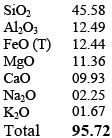Solution:
*Answer can only contain numeric values
QUESTION: 57

As shown in the following figure, a vertical well intersects the top and bottom of an inclined bed at 200 m and 410 m depths, respectively. If the true dip of the bed is 60o to the north, the true thickness of the bed is _______metres.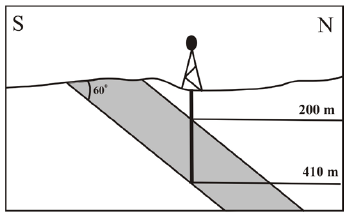Solution:
*Answer can only contain numeric values
QUESTION: 58

In the given geologic map, the horizontal lines are stratum contours. The throw on the fault (F-F) is _________meters.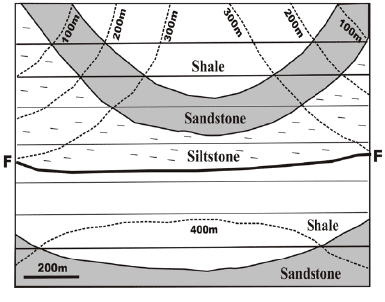Solution:
*Answer can only contain numeric values
QUESTION: 59

Two localities A and B on a continental plate as shown in the figure below, are separated by a distance of 80 km. The plate velocities measured at A and B are 4 cm/yr and 5 cm/yr, respectively.
Assuming no faulting in the area, the new distance between A and B will be_________ km in one million years. Give answer in two decimal places.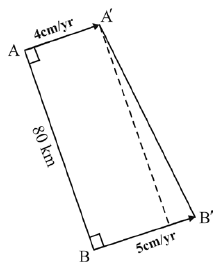Solution:
*Answer can only contain numeric values
QUESTION: 60

In the following schematic diagram, the aluminosilicate triple point is located at the pressure of 4.50 kbar and temperature of 823oK. If the reaction andalusite = sillimanite has negative slope with a value of –18.22 bar/oK, the pressure of the reaction at 923oK is ________kbar (give answer in two decimal places).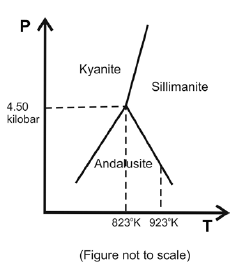Solution: